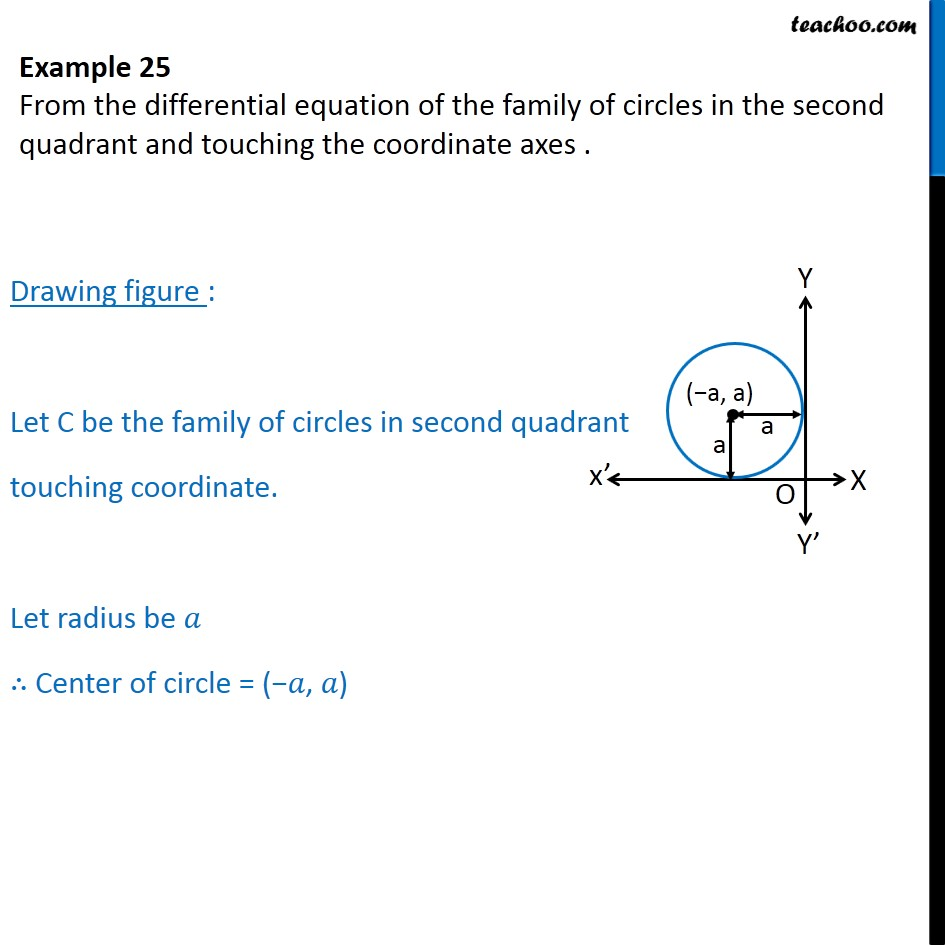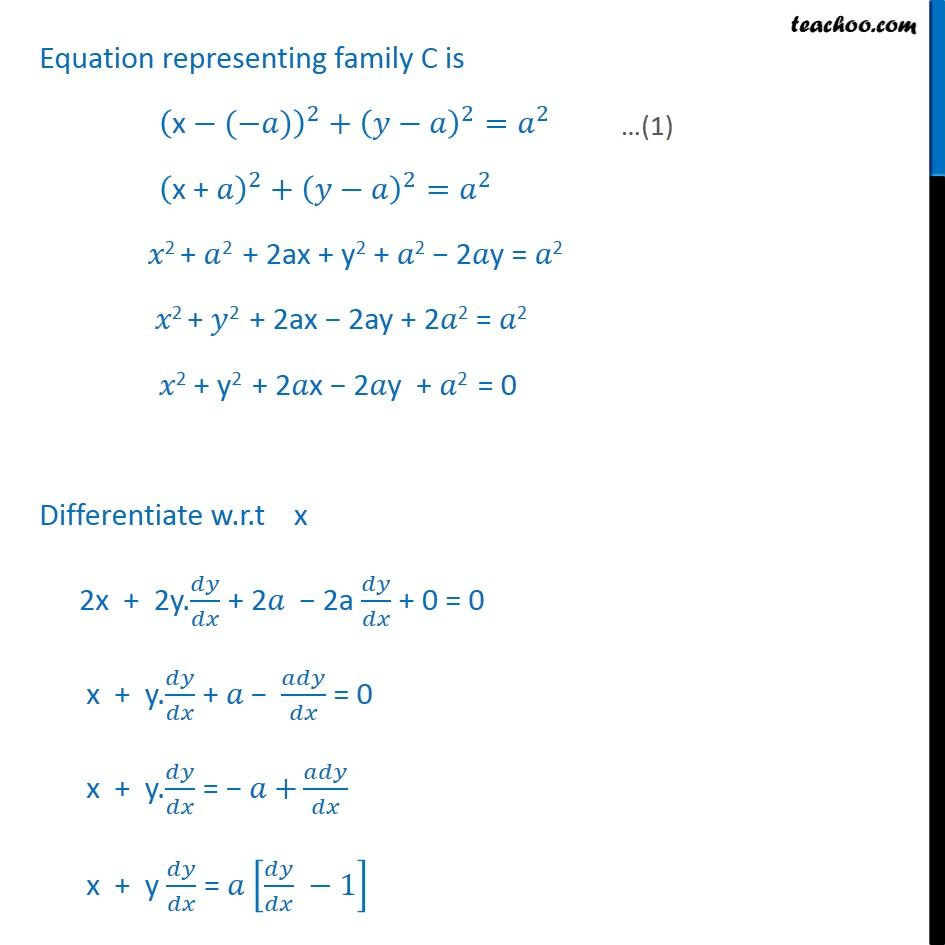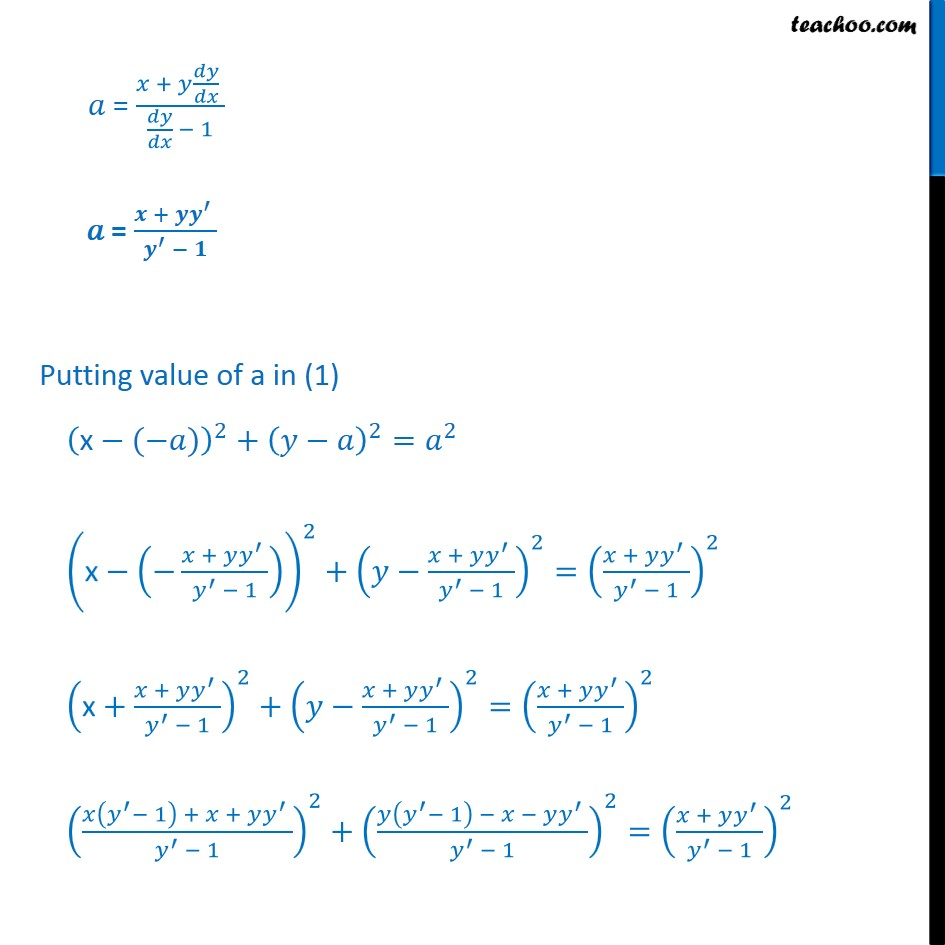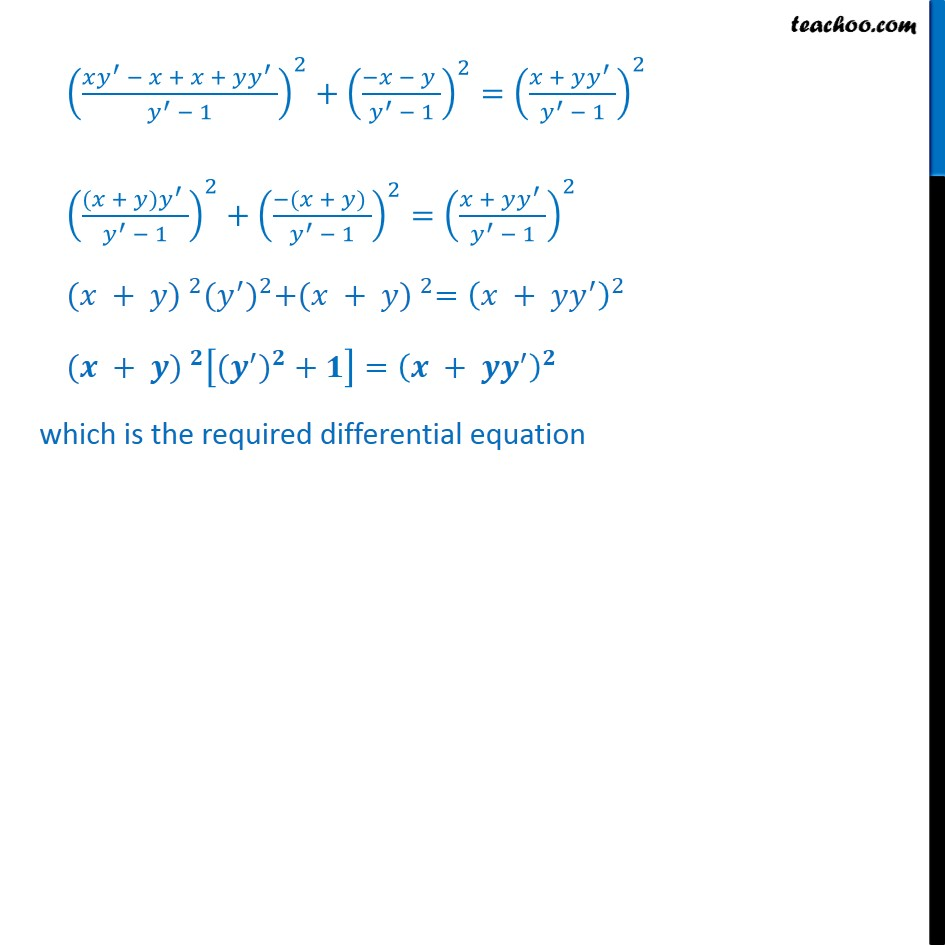Examples

Chapter 9 Class 12 Differential Equations
Serial order wiseLearn in your speed, with individual attention - Teachoo Maths 1-on-1 Class

### Transcript

Question 6 From the differential equation of the family of circles in the second quadrant and touching the coordinate axes . Drawing figure : Let C be the family of circles in second quadrant touching coordinate. Let radius be 𝑎 ∴ Center of circle = (−𝑎, 𝑎) Equation representing family C is x−(−𝑎)﷯﷮2﷯+ 𝑦−𝑎﷯﷮2﷯= 𝑎﷮2﷯ x + 𝑎﷯﷮2﷯+ 𝑦−𝑎﷯﷮2﷯= 𝑎﷮2﷯ 𝑥2 + 𝑎2 + 2ax + y2 + 𝑎2 − 2𝑎y = 𝑎2 𝑥2 + 𝑦2 + 2ax − 2ay + 2𝑎2 = 𝑎2 𝑥2 + y2 + 2𝑎x − 2𝑎y + 𝑎2 = 0 Differentiate w.r.t x 2x + 2y. 𝑑𝑦﷮𝑑𝑥﷯ + 2𝑎 − 2a 𝑑𝑦﷮𝑑𝑥﷯ + 0 = 0 x + y. 𝑑𝑦﷮𝑑𝑥﷯ + 𝑎 − 𝑎𝑑𝑦﷮𝑑𝑥﷯ = 0 x + y. 𝑑𝑦﷮𝑑𝑥﷯ = − 𝑎 + 𝑎𝑑𝑦﷮𝑑𝑥﷯ x + y 𝑑𝑦﷮𝑑𝑥﷯ = 𝑎 𝑑𝑦﷮𝑑𝑥﷯ −1﷯ 𝑎 = 𝑥 + 𝑦 𝑑𝑦﷮𝑑𝑥﷯ ﷮ 𝑑𝑦﷮𝑑𝑥﷯ − 1﷯ 𝑎 = 𝒙 + 𝒚 𝒚﷮′﷯ ﷮ 𝒚﷮′﷯ − 𝟏﷯ Putting value of a in (1) x−(−𝑎)﷯﷮2﷯+ 𝑦−𝑎﷯﷮2﷯= 𝑎﷮2﷯ x− − 𝑥 + 𝑦 𝑦﷮′﷯ ﷮ 𝑦﷮′﷯ − 1﷯﷯﷯﷮2﷯+ 𝑦− 𝑥 + 𝑦 𝑦﷮′﷯ ﷮ 𝑦﷮′﷯ − 1﷯﷯﷮2﷯= 𝑥 + 𝑦 𝑦﷮′﷯ ﷮ 𝑦﷮′﷯ − 1﷯﷯﷮2﷯ x+ 𝑥 + 𝑦 𝑦﷮′﷯ ﷮ 𝑦﷮′﷯ − 1﷯﷯﷮2﷯+ 𝑦− 𝑥 + 𝑦 𝑦﷮′﷯ ﷮ 𝑦﷮′﷯ − 1﷯﷯﷮2﷯= 𝑥 + 𝑦 𝑦﷮′﷯ ﷮ 𝑦﷮′﷯ − 1﷯﷯﷮2﷯ 𝑥 𝑦﷮′﷯− 1﷯ + 𝑥 + 𝑦 𝑦﷮′﷯ ﷮ 𝑦﷮′﷯ − 1﷯﷯﷮2﷯+ 𝑦 𝑦﷮′﷯− 1﷯ − 𝑥 − 𝑦 𝑦﷮′﷯ ﷮ 𝑦﷮′﷯ − 1﷯﷯﷮2﷯= 𝑥 + 𝑦 𝑦﷮′﷯ ﷮ 𝑦﷮′﷯ − 1﷯﷯﷮2﷯ 𝑥 𝑦﷮′﷯ − 𝑥 + 𝑥 + 𝑦 𝑦﷮′﷯ ﷮ 𝑦﷮′﷯ − 1﷯﷯﷮2﷯+ −𝑥 − 𝑦 ﷮ 𝑦﷮′﷯ − 1﷯﷯﷮2﷯= 𝑥 + 𝑦 𝑦﷮′﷯ ﷮ 𝑦﷮′﷯ − 1﷯﷯﷮2﷯ (𝑥 + 𝑦) 𝑦﷮′﷯ ﷮ 𝑦﷮′﷯ − 1﷯﷯﷮2﷯+ −(𝑥 + 𝑦) ﷮ 𝑦﷮′﷯ − 1﷯﷯﷮2﷯= 𝑥 + 𝑦 𝑦﷮′﷯ ﷮ 𝑦﷮′﷯ − 1﷯﷯﷮2﷯ (𝑥 + 𝑦) ﷮2﷯ ( 𝑦﷮′﷯)﷮2﷯+ (𝑥 + 𝑦) ﷮2﷯= 𝑥 + 𝑦 𝑦﷮′﷯﷯﷮2﷯ (𝒙 + 𝒚) ﷮𝟐﷯ ( 𝒚﷮′﷯)﷮𝟐﷯ + 𝟏﷯= 𝒙 + 𝒚 𝒚﷮′﷯﷯﷮𝟐﷯ which is the required differential equation